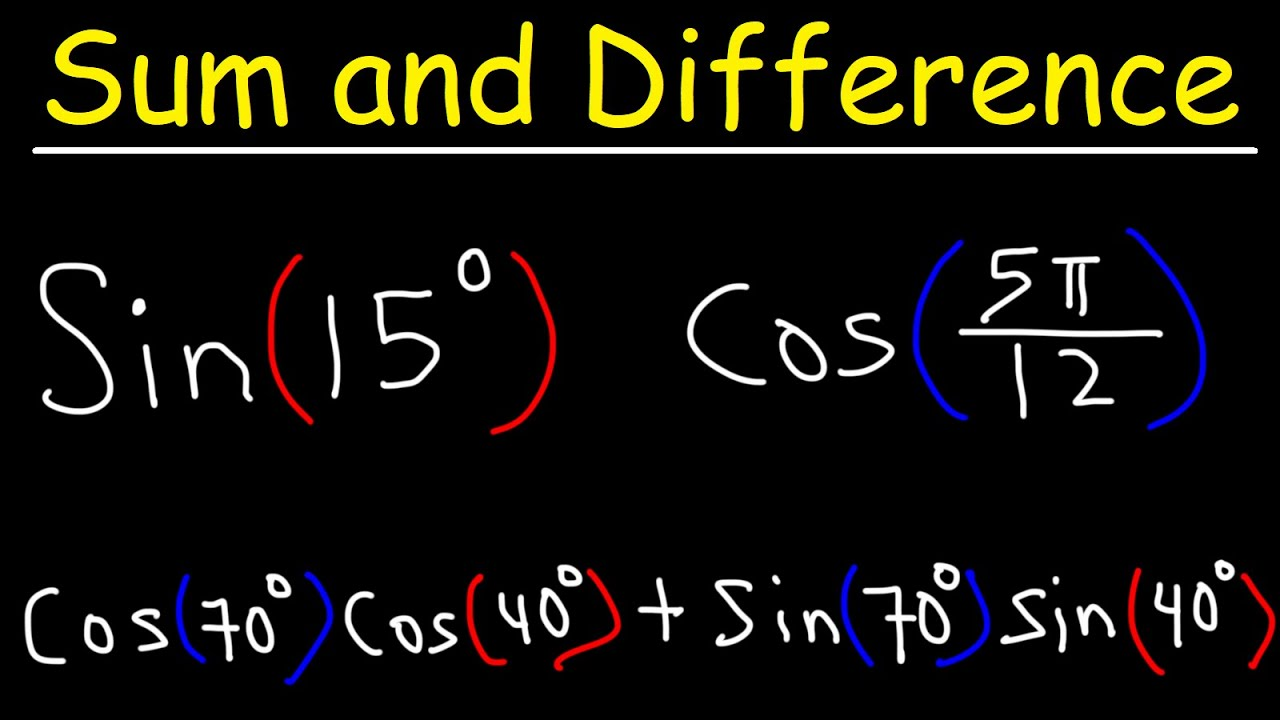1625514300

# Sum and Difference Identities of Sine and Cosine

This trigonometry video tutorial explains how to find the exact of trigonometric expressions with angles in radians and degrees using the sum and difference identities & formulas of sine and cosine. This lesson contains plenty of examples and practice problems that will help you to get a thorough understanding of this material.

Full 1 Hour Video:
https://www.patreon.com/MathScienceTutor

#developer